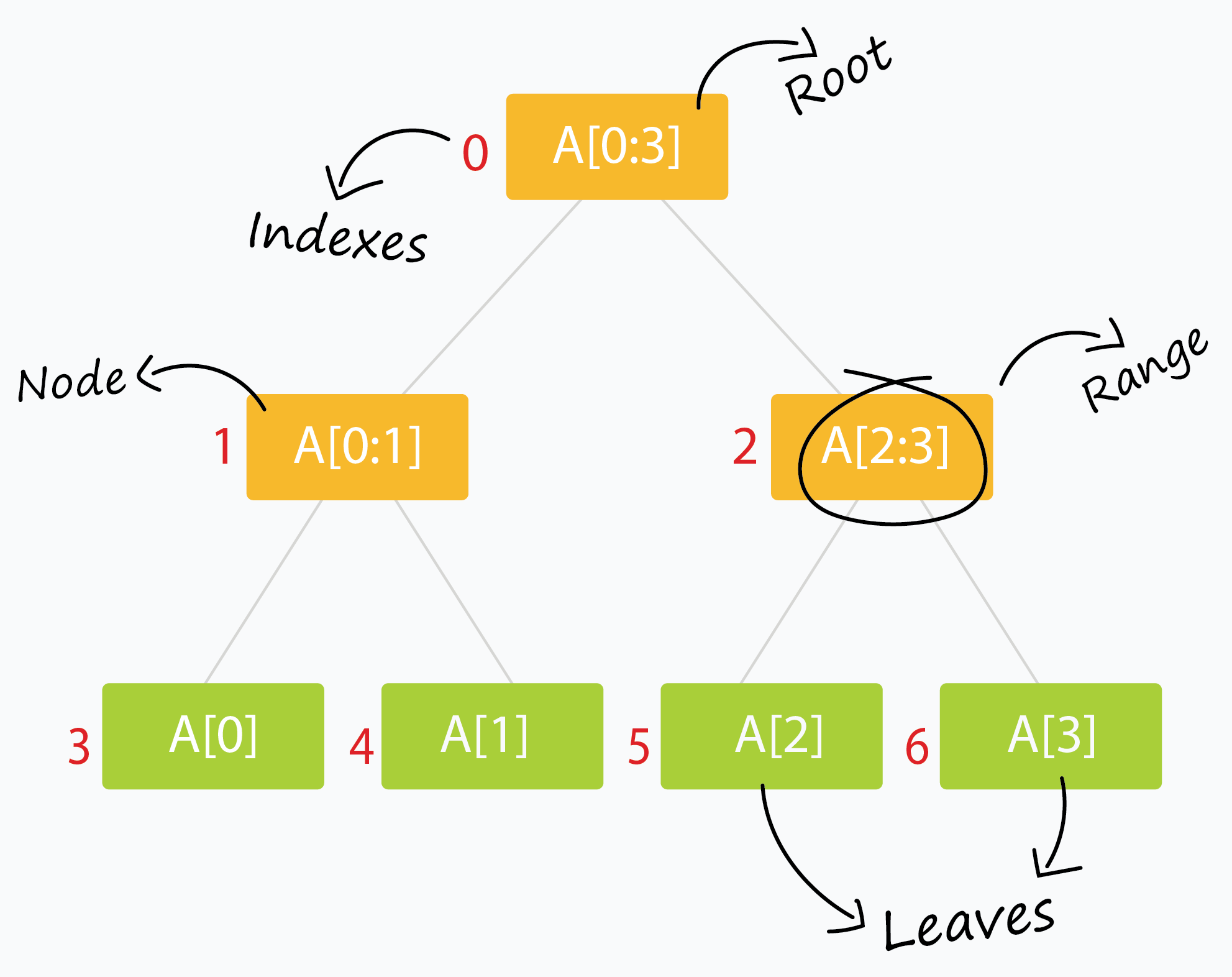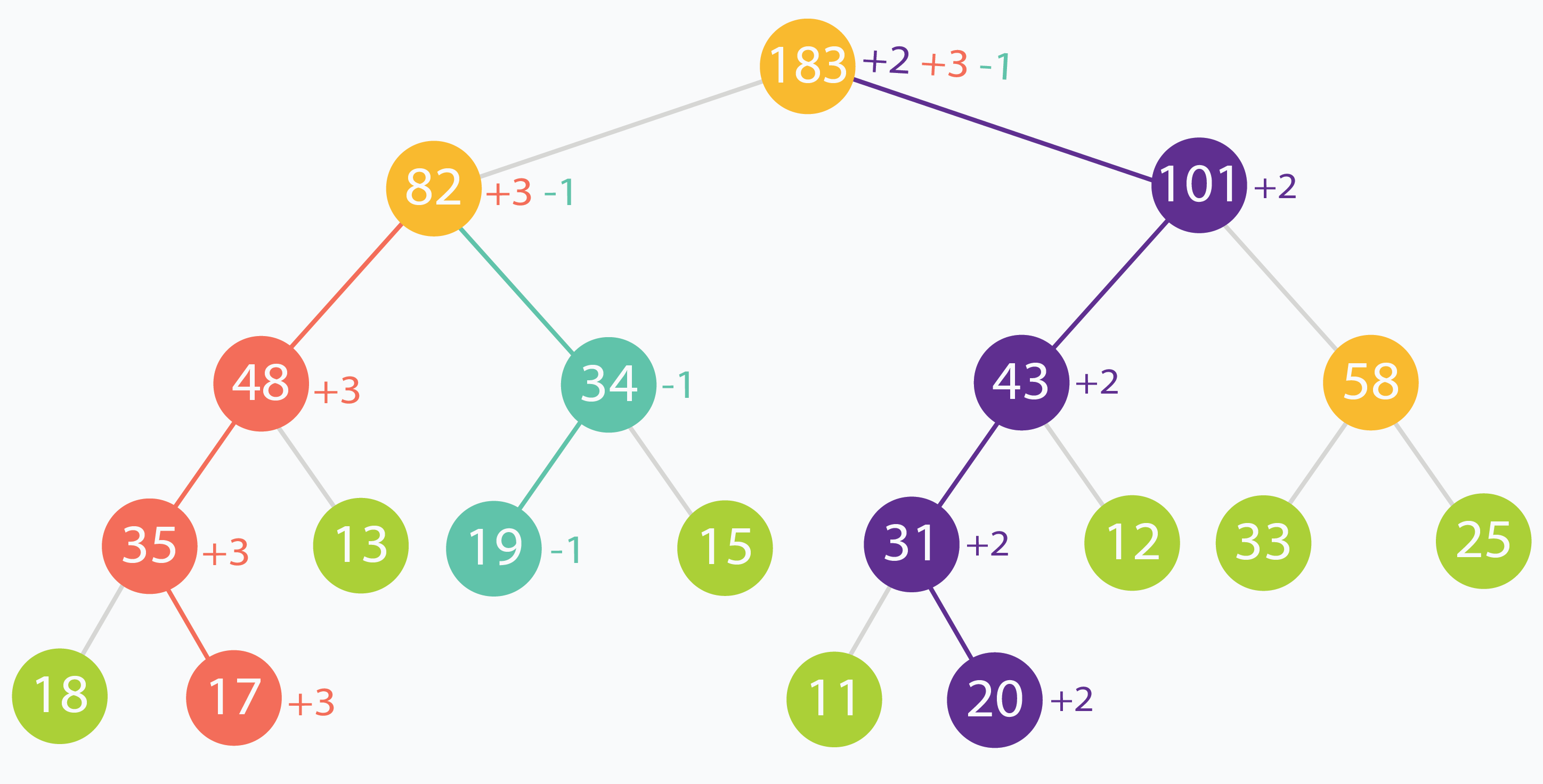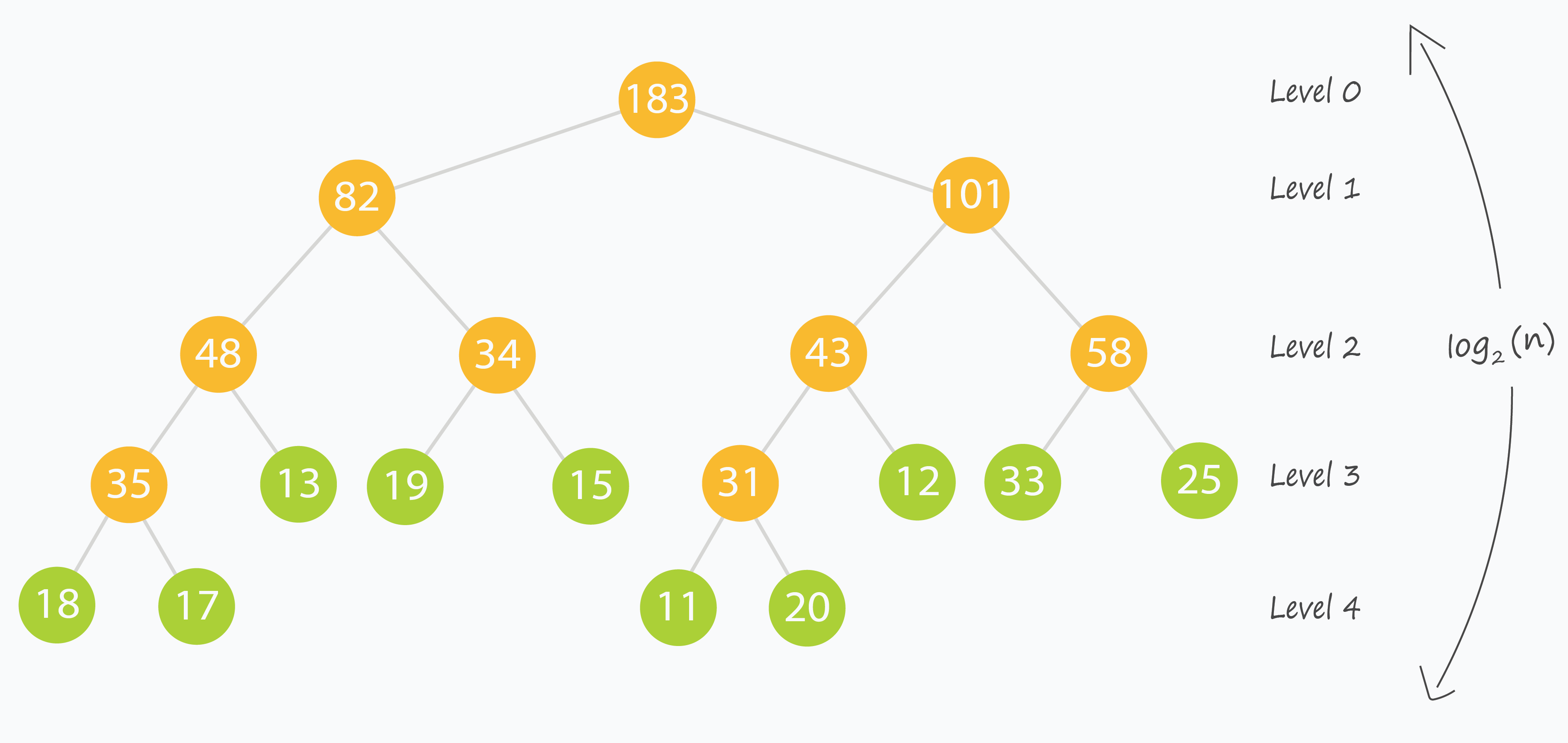## Recursive Approach to Segment Trees

### Brief Introduction

#### What is a Segment Tree?

A segment tree is a binary tree where each node represents an interval. Generally a node would store one or more properties of an interval which can be queried later.#### Why do we require it? (or What's the point of this?)

Many problems require that we give results based on query over a range or segment of available data. This can be a tedious and slow process, especially if the number of queries is large and repetitive. A segment tree let's us process such queries efficiently in logarithmic order of time.

Segment Trees have applications in areas of computational geometry and geographic information systems. For example, we may have a large number of points in space at certain distances from a central reference/origin point. Suppose we have to lookup the points which are in a certain range of distances from our origin. An ordinary lookup table would require a linear scan over all the possible points or all possible distances (think hash-maps). Segment Trees lets us achieve this in logarithmic time with much less space cost. Such a problem is called Planar Range Searching. Solving such problems efficiently is critical, especially when dealing with dynamic data which changes fast and unpredictably (for example, a radar system for air traffic.)

We will solve the Range Sum Query problem later in this editorial as an example of how Segment Trees help us save loads on runtime costs.We will use the above tree as a practical example of what a Range Sum Query segment tree looks and behaves like.

#### How do we make one?

Let our data be in an array arr[] of size .

1. The root of our segment tree typically represents the entire interval of data we are interested in. This would be arr[0:n-1].
2. Each leaf of the tree represents a range comprising of just a single element. Thus the leaves represent arr, arr and so on till arr[n-1].
3. The internal nodes of the tree would represent the merged or union result of their children nodes.
4. Each of the children nodes could represent approximately half of the range represented by their parent.

A segment tree for an element range can be comfortably represented using an array of size . (Stack Overflow has a good discussion as to why. If you are not convinced, fret not. We will discuss it later on.)

But how? The idea is simple: A node at index can have two children at indexes and .Segment trees are very intuitive and easy to use when built recursively.

### Recursive methods for Segment Trees

We will use the array tree[] to store the nodes of our segment tree (initialized to all zeros). The following scheme (0 - based indexing) is used:

• The node of the tree is at index . Thus tree is the root of our tree.
• The children of tree[i] are stored at tree[2*i+1] and tree[2*i+2].
• We will pad our arr[] with extra 0 or null values so that (where is the final length of arr[] and is a non negative integer.)

Do we actually need to pad arr[] with zeros? No, not really. Just ensure that tree[] is large enough and always zero-initialized and you don't need to worry about extra leaf nodes not being processed.

• The leaves of the tree occur at indexes to .

What if we started by storing the node of tree at index instead of index ? How would the positions of related nodes change? For starters, the children for node at index will now be located at indexes and . Can you determine which indexes the leaves will be stored at?We also require only three kinds of methods:

#### 1. Build the tree from the original data.

The method builds the entire tree in a bottom up fashion. When the condition is satisfied, we are left with a range comprising of just a single element (which happens to be arr[lo]). This constitutes a leaf of the tree. The rest of the nodes are built by merging the results of their two children. treeIndex is the index of the current node of the segment tree which is being processed.For example, the tree above is made from the input array: (which we will use throughout this tutorial) Can you guess what the merge operation is in this example? After building the tree, the tree[] array looks like: Notice the the groups of zeros near the end of the tree[] array? Those are null values we used as padding to ensure a complete binary tree is formed (since we only had leaf elements. Had we had, say, leaf elements, we wouldn't need any null elements. Can you prove why?)

NOTE: The merge operation varies from problem to problem. You should closely think of what to store in a node of the segment tree and how two nodes will merge to provide a result before you even start building a segment tree.

#### 2. Read/Query on an interval or segment of the data.

The method returns a result when the queried range matches exactly with the range represented by a current node. Else it digs deeper into the tree to find nodes which match a portion of the node exactly.

This is where the beauty of the segment tree lies.In the above example, we are trying to find the sum of the elements in the range . No segment completely represents the range . However we can see that can be built up using the ranges and As a quick verification, we can see that sum of input elements at indexes is . The sum of node values for the nodes representing ranges and are

#### 3. Update the value of an element.

This is similar to buildSegTree. We update the value of the leaf node of our tree which corresponds to the updated element. Later the changes are propagated through the upper levels of the tree straight to the root.In this example, element at indexes (in original input data) and are incremented by and respectively. You can see how the changes propagate up the tree, all through to the root.

### Complexity Analysis

Let's take a look at the build process. We visit each leaf of the segment tree (corresponding to each element in our array arr[]). That makes leaves. Also there will be internal nodes. So we process about nodes. This makes the build process run in linear complexity.

The update process discards half of the range for every level of recursion to reach the appropriate leaf in the tree. This is similar to binary search and takes logarithmic time. After the leaf is updated, its direct ancestors at each level of the tree are updated. This takes time linear to height of the tree.

The read/query process traverses depth-first through the tree looking for node(s) that match exactly with the queried range. At best, we query for the entire range and get our result from the root of the segment tree itself. At worst, we query for a interval/range of size (which corresponds to a single element), and we end up traversing through the height of the tree. This takes time linear to height of the tree.This is the time to revisit something said before:

A segment tree for an element range can be comfortably represented using an array of size .

This ensures that we build our segment tree as a complete binary tree, which in turn ensures that the height of the tree is upper-bounded by the logarithm of the size of our input.

Voila! Both the read and update queries now take logarithmic time, which is what we desired.

## Range Sum QueriesThe Range Sum Query problem is a subset of the Range Query class of problems. Given an array or sequence of data elements, one is required to process read and update queries which consist of ranges of elements. Segment Trees (along with other Interval-based data structures like the Binary Indexed Tree (a.k.a. Fenwick Tree)) are used to solve this class of problems reasonably fast for practical usage.

The Range Sum Query problem specifically deals with the sum of elements in the queried range. Many variations of the problem exist, including for immutable data, mutable data, multiple updates, single query and multiple updates, multiple queries (each being very costly in terms of computation).

A sample solution solves the Range Sum Query problem for mutable arrays efficiently through the use of a recursive segment tree (pretty much like the one we just discussed.) The merge operation in this case is simply taking the sum of the two nodes (since each node stores the sum of the range it represents.)

## Lazy Propagation

### Motivation

Till now we have been updating single elements only. That happens in logarithmic time and it's pretty efficient.

But what if we had to update a range of elements? By our current method, each of the elements would have to be updated independently, each incurring some run time cost.

The construction of a tree poses another issue called ancestral locality. Ancestors of adjacent leaves are guaranteed to be common at some levels of the tree. Updating each of these leaves individually would mean that we process their common ancestors multiple times. What if we could reduce this repetitive computation?In the above example, the root is updated thrice and the node numbered is updated twice. This is because, at some level of the tree, the changes propagated from different leaves will meet.

A third kind of problem is when queried ranges do not contain frequently updated elements. We might be wasting valuable time updating nodes which are rarely going to be accessed/read.

Using Lazy Propagation allows us to overcome all of these problems by reducing wasteful computations and processing nodes on-demand.

### How do we use it?

As the name suggests, we update nodes lazily. In short, we try to postpone updating descendants of a node, until the descendants themselves need to be accessed.

For the purpose of applying it to the Range Sum Query problem, we assume that the update operation on a range, increments each element in the range by some amount val.

We use another array lazy[] which is the same size as our segment tree array tree[] to represent a lazy node. lazy[i] holds the amount by which the node tree[i] needs to be incremented, when that node is finally accessed or queried. When lazy[i] is zero, it means that node tree[i] is not lazy and has no pending updates.

#### 1. Updating a range lazily

This is a three step process:

1. Normalize the current node. This is done by removing laziness. We simple increment the current node by appropriate amount to remove it's laziness. Then we mark its children to be lazy as the descendants haven't been processed yet.
2. Apply the current update operation to the current node if the current segment lies inside the update range.
3. Recurse for the children as you would normally to find appropriate segments to update.

#### 2. Querying a lazily propagated tree

This is a two step process:

1. Normalize the current node by removing laziness. This step is the same as the update step.
2. Recurse for the children as you would normally to find appropriate segments which fit in queried range.

NOTE: The following lines: are specific to the Range Sum Query problem. Different problems may have different updating and merging schemes. In this case, updates are increments of and nodes contain the sum of the elements of range/segment they represent.

## Bonus

• A quick cheat-sheet I recommend is located here.
• VisuAlgo has an awesome visualizer for segment trees here. Put in the example data in this tutorial to see the segment tree operations in action!

Tutorial written by @babhishek21.